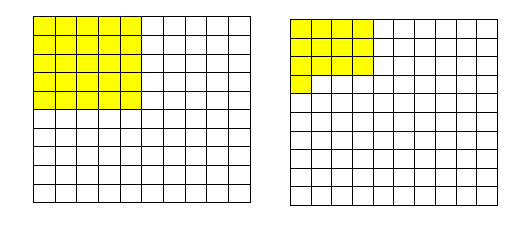# Decimal Number Comparison - Through Graphical And Analytical Method

The concept of the decimal is used in each and every field of mathematics. For example measurement of weight, length, money etc. And in each of these sections, there are instances where we need to compare two numbers or quantities. Let us say, we go to a local market to purchase pulse, where one of the vendors is selling the pulse at Rs 35.50/kg and the other is selling it at Rs 35.70/kg. In order to judge which shop we should buy the pulse from, we need to compare both the prices. These prices, as we saw, are in decimal numbers, so it is necessary to learn how decimal numbers are compared.

## Comparing Decimal Numbers

There are two methods involved in the decimal number comparisons. Let us discuss the two different methods here in detail.

### Decimal Comparison Method 1

Example: Comparing 0.25 and 0.13

• We take a piece of paper and divide it into rows and columns equivalent to the number of places after decimal we are dealing with. For example, if we are dealing with numbers with one place after the decimal, we draw 10 blocks, 5*2 or 10*1; for two places after the decimal we draw 100 blocks with 10 rows and 10 columns.
• Since the decimals we are dealing with have maximum two places after the decimal, we draw a 10*10 box.
• Upon conversion into fraction, we can see, 0.25 = 25/100, so we fill 25 blocks out of 100 and for 0.13 we fill 13 blocks out of 100.• By observation, we can say that 0.25 is greater than 0.13 as the coloured region in 0.25 is greater than 0.13.

The above method is time taking and not suitable for numbers with more than two places after the decimal. For such numbers method, 2 is beneficial and efficient.

### Decimal Comparison Method 2

Example :  Comparing 7.345 and 7.38

• The whole number present before the decimal is compared first. The number with a greater whole number is considered bigger. In this example, the whole number present before the decimal is the same for both the cases.
• The digit at the tenth place is compared. The number with a bigger digit at the tenth place is considered greater. In this example, both the numbers have the same digit in tenth place.
• The digit at the hundredth place after the decimal is compared. In this example, the digit at the hundredths place for the number 7.38 is greater than that for 7.345. Hence, the number 7.38 is greater than 7.345.

## Video Lesson on Numbers### Decimal Number Comparison Examples

 Let’s Work Out: Example: Compare the given decimal numbers and state whether the given decimals are greater than, less than or equal to each other. (a) 4.1245 or 4.124 (b) 7.524 or 7.5240 (c) 254.913 or 254.15 (d) 1.102 or 1.120 Solution: (a) 4.1245 or 4.124 4.125 > 4.124 (b) 7.524 or 7.5240 7.524 = 7.5240 (c) 254.913 or 254.15 254.913 > 254.15 (d) 1.102 or 1.120 1.102 <.1.120